Courses

# Measurement of Angles with Examples Mathematics Notes | EduRev

## Mathematics : Measurement of Angles with Examples Mathematics Notes | EduRev

The document Measurement of Angles with Examples Mathematics Notes | EduRev is a part of the Mathematics Course Additional Topics for IIT JAM Mathematics.
All you need of Mathematics at this link: Mathematics

The concept of angle
The concept of angle is one of the most important concepts in geometry. The concepts of equality, sums, and differences of angles are important and used throughout geometry, but the subject of trigonometry is based on the measurement of angles.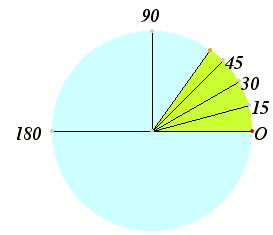There are two commonly used units of measurement for angles. The more familiar unit of measurement is that of degrees. A circle is divided into 360 equal degrees, so that a right angle is 90°. For the time being, we’ll only consider angles between 0° and 360°, but later, in the section on trigonometric functions, we’ll consider angles greater than 360° and negative angles.
Degrees may be further divided into minutes and seconds, but that division is not as universal as it used to be. Each degree is divided into 60 equal parts called minutes. So seven and a half degrees can be called 7 degrees and 30 minutes, written 7° 30'. Each minute is further divided into 60 equal parts called seconds, and, for instance, 2 degrees 5 minutes 30 seconds is written 2° 5' 30". The division of degrees into minutes and seconds of angle is analogous to the division of hours into minutes and seconds of time.
Parts of a degree are now usually referred to decimally. For instance seven and a half degrees is now usually written 7.5°.
When a single angle is drawn on a xy-plane for analysis, we’ll draw it in standard position with the vertex at the origin (0,0), one side of the angle along the x-axis, and the other side above the x-axis.

The other common measurement for angles is radians. For this measurement, consider the unit circle (a circle of radius 1) whose center is the vertex of the angle in question. Then the angle cuts off an arc of the circle, and the length of that arc is the radian measure of the angle. It is easy to convert between degree measurement and radian measurement. The circumference of the entire circle is 2π, so it follows that 360° equals 2π radians. Hence,
and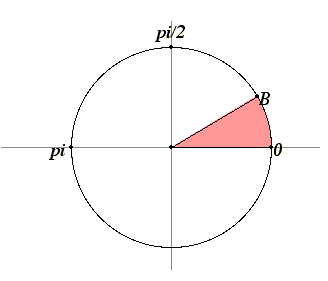Most calculators can be set to use angles measured with either degrees or radians. Be sure you know what mode your calculator is using.

Short note on the history of radians
Although the word “radian” was coined by Thomas Muir and/or James Thompson about 1870, mathematicians had been measuring angles that way for a long time. For instance, Leonhard Euler (1707–1783) in his Elements of Algebra explicitly said to measure angles by the length of the arc cut off in the unit circle. That was necessary to give his famous formula involving complex numbers that relates the sign and cosine functions to the exponential function
e = cos θ + i sin θ
where θ is what was later called the radian measurment of the angle. Unfortunately, an explanation of this formula is well beyond the scope of these notes.

An alternate definition of radians is sometimes given as a ratio. Instead of taking the unit circle with center at the vertex of the angle θ, take any circle with center at the vertex of the angle. Then the radian measure of the angle is the ratio of the length of the subtended arc to the radius r of the circle. For instance, if the length of the arc is 3 and the radius of the circle is 2, then the radian measure is 1.5.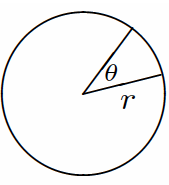The reason that this definition works is that the length of the subtended arc is proportional to the radius of the circle. In particular, the definition in terms of a ratio gives the same figure as that given above using the unit circle. This alternate definition is more useful, however, since you can use it to relate lengths of arcs to angles. The length of an arc is is the radius r times the angle θ where the angle is measured in radians.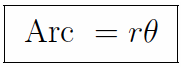For instance, an arc of θ = 0.3 radians in a circle of radius r = 4 has length 0.3 times 4, that is, 1.2.

A sector of a circle is that part of a circle bounded by two radii and the arc of the circle that joins their ends. The area of this sector is easy to compute from the radius r of the circle and the angle θ beween the radii when it’s measured in radians. Since the area of the whole circle is πr2, and the sector is to the whole circle as the angle θ is to 2π, therefore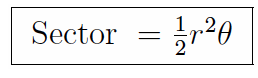Common angles
Below is a table of common angles in both degree measurement and radian measurement. Note that the radian measurement is given in terms of π. It could, of course, be given decimally, but radian measurement often appears with a factor of π .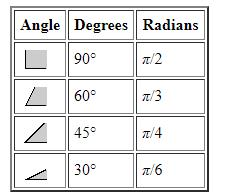Exercises
Edwin S. Crawley wrote a book One Thousand Exercises in Plane and Spherical Trigonometry, University of Pennsylvania, Philadelphia, 1914. The problems in this short course are taken from that text (but not all 1000 of them!) He gave his problems with up to five digits of accuracy, so students had to work some time to solve them, and they used tables of logarithms to help in multiplication and division. Students had to be able to use the sine-cosine table, the tangent table, the logarithm table, the log-sin-cos table, and the log-tan table. Now we can use calculators! That means you can concentrate on the concepts and not on laborious computations.
Crawley didn’t used decimal notation for fractions of a degree, but minutes and seconds.
Each set of exercises includes first the statements of the exercises, second some hints to solve the exercises, and third the answer to the exercises.
1. Express the following angles in radians.
(a). 12 degrees, 28 minutes, that is, 12° 28'.
(b). 36° 12'.

2. Reduce the following numbers of radians to degrees, minutes, and seconds.
(a). 0.47623.
(b). 0.25412.

3. Given the angle a and the radius r, to find the length of the subtending arc.
(a). a = 0° 17' 48", r = 6.2935.
(b). a = 121° 6' 18", r = 0.2163.

4. Given the length of the arc l and the radius r, to find the angle subtended at the center.
(a). l = .16296, r = 12.587.
(b). l = 1.3672, r = 1.2978.

5. Given the length of the arc l and the angle a which it subtends at the center, to find the radius.
(a). a = 0° 44' 30", l = .032592.
(b). a = 60° 21' 6", l = .4572.

6. Find the length to the nearest inch of a circular arc of 11 degrees 48.3 minutes if the radius is 3200 feet.

7. A railroad curve forms a circular arc of 9 degrees 36.7 minutes, the radius to the center line of the track being 2100 feet. If the gauge is 5 feet, find the difference in length of the two rails to the nearest half-inch.

9. How much does one change latitude by walking due north one mile, assuming the earth to be a sphere of radius 3956 miles?

10. Compute the length in feet of one minute of arc on a great circle of the earth. How long is the length of one second of arc?

14. On a circle of radius 5.782 meters the length of an arc is 1.742 meters. What angle does it subtend at the center?
23. A balloon known to be 50 feet in diameter subtends at the eye an angle of 8 1/2 minutes. How far away is it?

Hints
1. To convert degrees to radians, first convert the number of degrees, minutes, and seconds to decimal form. Divide the number of minutes by 60 and add to the number of degrees. So, for example, 12° 28' is 12 + 28/60 which equals 12.467°. Next multiply by π and divide by 180 to get the angle in radians.
2. Conversely, to convert radians to degrees divide by π and multiply by 180. So, 0.47623 divided by π and multiplied by 180 gives 27.286°. You can convert the fractions of a degree to minutes and seconds as follows. Multiply the fraction by 60 to get the number of minutes. Here, 0.286 times 60 equals 17.16, so the angle could be written as 27° 17.16'. Then take any fraction of a minute that remains and multiply by 60 again to get the number of seconds. Here, 0.16 times 60 equals about 10, so the angle can also be written as 27° 17' 10".
3. In order to find the length of the arc, first convert the angle to radians. For 3(a), 0°17'48" is 0.0051778 radians. Then multiply by the radius to find the length of the arc.
4. To find the angle, divide by the radius. That gives you the angle in radians. That can be converted to degrees to get Crawley’s answers.
5. As mentioned above, radian measure times radius = arc length, so, using the letters for this problem, ar = l, but a needs to be converted from degree measurement to radian measurement first. So, to find the radius r, first convert the angle a to radians, then divide that into the length l of the arc.
7. It helps to draw the figure. The radius to the outer rail is 2102.5 while the radius to the inner rail is 2097.5.
9. You’ve got a circle of radius 3956 miles and an arc of that circle of length 1 mile. What is the angle in degrees? (The mean radius of the earth was known fairly accurately in 1914. See if you can find out what Eratosthenes thought the radius of the earth was back in the third century B.C.E.)
10. A minute of arc is 1/60 of a degree. Convert to radians. The radius is 3956. What is the length of the arc?
14. Since the length of the arc equals radius times the angle in radians, it follows that the angle in radians equals the length of the arc divided by the radius. It’s easy to convert radians to degrees.
23. Imagine that the diameter of the balloon is a part of an arc of a circle with you at the center. (It isn’t exactly part of the arc, but it’s pretty close.) That arc is 50 feet long. You know the angle, so what is the radius of that circle?

1. (a). 0.2176.
(b). 0.6318.
2. (a). 27° 17' 10".
(b). 14.56° = 14°33.6' = 14°33'36".
3. (a). 0.03259
(b). 2.1137 times 0.2163 equals 0.4572.
4. (a). 0.16296/12.587 = 0.012947 radians = 0° 44' 30".
(b). 1.3672/1.2978 = 1.0535 radians = 60.360° = 60° 21.6' = 60° 21' 35".
5. (a). l/a = .032592/.01294 = 2.518.
(b). l/a = .4572/1.0533 = .4340.
6. ra = (3200') (0.20604) = 659.31' = 659' 4".
7. The angle a = 0.16776 radians. The difference in the lengths is 2102.5a – 1997.5a which is 5a. Thus, the answer is 0.84 feet, which to the nearest inch is 10 inches.
9. Angle = 1/3956 = 0.0002528 radians = 0.01448° = 0.8690' = 52.14".
10. One minute = 0.0002909 radians. 1.15075 miles = 6076 feet. Therefore one second will correspond to 101.3 feet.
14. a = l/r = 1.742/5.782 = 0.3013 radians = 17.26° = 17°16'.
23. The angle a is 8.5', which is 0.00247 radians. So the radius is r = l/a = 50/0.00247 = 20222' = 3.83 miles, nearly four miles.

Crawley is careful to give his answers with about the same accuracy as the data in the questions. This is important, especially now that we have calculators. For example, in problem 1, the datum is 12°28', which has about four digits of accuracy, so the answer, 0.2176, should also be given with only four digits of accuracy. (Note that leading zeros don’t count in figuring digits of accuracy.) An answer of 0.21758438 suggests eight digits of accuracy, and that would be misleading as the given information wasn’t that accurate.
For another example, see problem 3(a). The data are 0°17'48" and 6.2935, with 4 and 5 digits of accuracy, respectively. The answer should, therefore, be given with only 4 digits of accuracy, since the answer can be no more accurate than the least accurate datum. Thus, the answer a calculator might give, namely 0.032586547 should be rounded to four digits (not including the leading zeros) to 0.03259.
Although final answers should be expressed with an appropriate number of digits of accuracy, you should still keep all the digits for intermediate computations.

Offer running on EduRev: Apply code STAYHOME200 to get INR 200 off on our premium plan EduRev Infinity!

40 docs

,

,

,

,

,

,

,

,

,

,

,

,

,

,

,

,

,

,

,

,

,

;